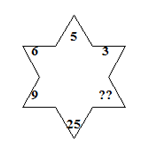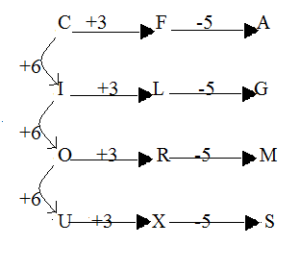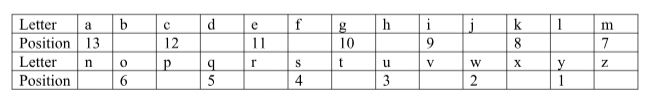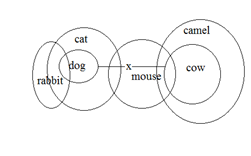# SSC CGL 2018 Practice Test Papers | Reasoning Ability (Day-15)

Dear Aspirants, Here we have given the Important SSC CGL Exam 2018 Practice Test Papers. Candidates those who are preparing for SSC CGL 2018 can practice these questions to get more confidence to Crack SSC CGL 2018 Examination.

[WpProQuiz 2878]

Click “Start Quiz” to attend these Questions and view Explanation

1) If ANT is coded as 34 and PEN is coded as 94 then how CAP is coded in that code?

a) 56

b) 19

c) 27

d) 36

2) Find the odd one.

a) B7I

b) K12W

c) H11S

d) F6K

3) CFA, ILG, ORM ____

a) RHS

b) UXS

c) DGA

d) TWR

1. If all the alphabets at even places are left out, the then remaining alphabets are numbered from 1,2,3….. then what is the position of M from right end?

a)11

b) 13

c) 6

d)7

1. Find the missing number?a) 6

b) 49

c) 27

d) 36

Direction : (6-9)Find the option which logically follows.

Statements:

All Dogs are Cat

Some cat is Mouse.

Some Dog are Rabbit.

No Dog is Cow.

Some Mouse is Cow.

All Cows are Camel.

a) If Conclusion I follows

b) If Conclusion II follows

c) If either A or B

d) If neither A or B

1. Conclusions:
1. Some Rabbit are Camel
2. Some Camel are cow
1. Conclusions:
1. Some cat is Mouse.
2. Some Dog are Mouse
1. Conclusions:
1. Some Rabbit are not Cow
2. No Rabbit is Cow
1. Conclusions:
1. Some Camel are Dog is a possibility.
2. No mouse is Camel.
1. Complete the series.

aaa_bcbb_c _d_ _cd_e

a) aabbcd

b) bbccdd

c) bbbcce

d) bbcccd

Explanation:

ANT=1*14+20=34

PEN= 16*5+14=94

CAP=3*1+16=19

Explanation:

B7I = 9 – 2 =7

K12W = 23 – 11 = 12

H11S= 19 – 8 = 11

F6K= 11 – 6 = 5

Explanation:Explanation:

The numbers in the lower half of the figure is square of the number diagonally opposite to it.

3==9

5==25

6==36.